# The PHREG Procedure

### Residuals

This section describes the computation of residuals (RESMART=, RESDEV=, RESSCH=, and RESSCO=) in the OUTPUT statement.

First, consider TIES=BRESLOW. Let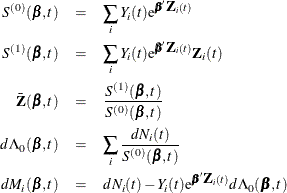The martingale residual at t is defined as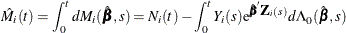Here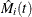estimates the difference over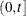between the observed number of events for the ith subject and a conditional expected number of events. The quantity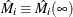is referred to as the martingale residual for the ith subject. When the counting process MODEL specification is used, the RESMART= variable contains the component (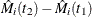) instead of the martingale residual at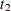. The martingale residual for a subject can be obtained by summing up these component residuals within the subject. For the Cox model with no time-dependent explanatory variables, the martingale residual for the ith subject with observation time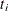and event status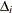is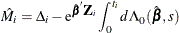The deviance residuals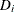are a transform of the martingale residuals: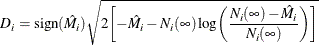The square root shrinks large negative martingale residuals, while the logarithmic transformation expands martingale residuals that are close to unity. As such, the deviance residuals are more symmetrically distributed around zero than the martingale residuals. For the Cox model, the deviance residual reduces to the form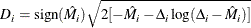When the counting process MODEL specification is used, values of the RESDEV= variable are set to missing because the deviance residuals can be calculated only on a per-subject basis.

The Schoenfeld (1982) residual vector is calculated on a per-event-time basis. At the jth event time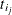of the ith subject, the Schoenfeld residual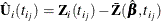is the difference between the ith subject covariate vector atand the average of the covariate vectors over the risk set at. Under the proportional hazards assumption, the Schoenfeld residuals have the sample path of a random walk; therefore, they are useful in assessing time trend or lack of proportionality. Harrell (1986) proposed a z-transform of the Pearson correlation between these residuals and the rank order of the failure time as a test statistic for nonproportional hazards. Therneau, Grambsch, and Fleming (1990) considered a Kolmogorov-type test based on the cumulative sum of the residuals.

The score process for the ith subject at time t is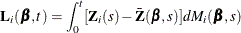The vector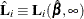is the score residual for the ith subject. When the counting process MODEL specification is used, the RESSCO= variables contain the components of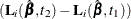instead of the score process at. The score residual for a subject can be obtained by summing up these component residuals within the subject.

The score residuals are a decomposition of the first partial derivative of the log likelihood. They are useful in assessing the influence of each subject on individual parameter estimates. They also play an important role in the computation of the robust sandwich variance estimators of Lin and Wei (1989) and Wei, Lin, and Weissfeld (1989).

For TIES=EFRON, the preceding computation is modified to comply with the Efron partial likelihood. For a given time t, let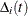=1 if the t is an event time of the ith subject and 0 otherwise. Let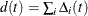, which is the number of subjects that have an event at t. For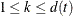, let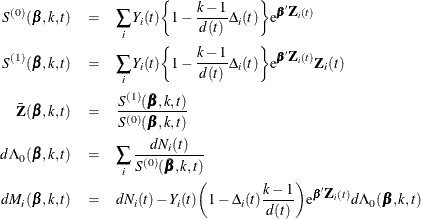The martingale residual at t for the ith subject is defined as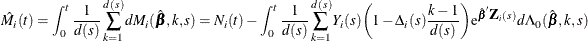Deviance residuals are computed by using the same transform on the corresponding martingale residuals as in TIES=BRESLOW.

The Schoenfeld residual vector for the ith subject at event timeis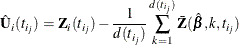The score process for the ith subject at time t is given by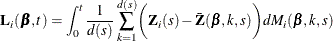For TIES=DISCRETE or TIES=EXACT, it is difficult to come up with modifications that are consistent with the corresponding partial likelihood. Residuals for these TIES= methods are computed by using the same formulas as in TIES=BRESLOW.